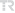## Power loss and voltage stability optimization with meta-heuristic algorithms in power system

PAMUKKALE UNIVERSITY JOURNAL OF ENGINEERING SCIENCES-PAMUKKALE UNIVERSITESI MUHENDISLIK BILIMLERI DERGISI, vol.27, no.2, pp.199-209, 2021 (ESCI)• Publication Type: Article / Article
• Volume: 27 Issue: 2
• Publication Date: 2021
• Doi Number: 10.5505/pajes.2020.84152
• Journal Name:
• Journal Indexes: Emerging Sources Citation Index (ESCI), TR DİZİN (ULAKBİM)
• Page Numbers: pp.199-209
• Keywords: Power Flow, Meta-heuristic algorithms, Voltage deviation, PARTICLE SWARM OPTIMIZATION, ALLOCATION, COLLAPSE
• Gazi University Affiliated: Yes

#### Abstract

Power flow, which is one of the most prominent problems in the field of power system, is the calculation of the voltage amplitudes and phase angles of each bus and the power losses by using the bus data with known steady state voltage amplitudes and power values. Increasing demand and the connection of decentralized energy sources to the power system at various points make more complicated power flow problem. The power flow problem is of great importance for both electricity generation and transmission. Planning new loads that can be connected to the system in the future and using the existing transmission lines at full capacity are based on the solution of the power flow problem. Power flow, which is a nonlinear problem, has traditionally been solved using numerical methods such as Newton-Raphson and Gauss Seidel. However, the success of classical solution algorithms decreases depending on the conditions of the power system. Meta-heuristic optimization techniques and search algorithms developed in recent years show that better results can be obtained in solving the power flow problem. In this study, Artificial Bee Colony (ABC), Gray Wolf (GWO), Particle Swarm Optimization (PSO) and Newton Raphson algorithms have been applied to optimize the power flow problem in the IEEE-14 bus test power system created using Matlab software. The performance of the algorithms has been compared by considering the voltage amplitudes, voltage deviation, phase angles, power losses and calculation times obtained from the model power system.